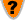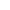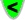Is the Big Bang theory correct?

 Q.  Is the Big Bang theory correct?A.  No. The Big Bang theory contains a number of wrong assumptions, and contravenes a basic law of Physics, the Law of Conservation of Mass/Energy.Q.  What does "Conservation of Mass/Energy" mean, and how is it not obeyed?A.  It means that any form of energy or mass can potentially be transformed into another form of mass or energy, but that the total amount of mass/energy remains the same.Q.  Is any case known of this Law not being obeyed?A.  Not in the real Universe, maybe in magic or myth. As Big Bang theory depicts a scenario where this Law is not obeyed, it is just another Creation myth.Q.  Where is there evidence that the Big Bang theory is incorrect?